# Gravimetric Analysis Introduction 1 Gravimetric Analysis i A

• Slides: 22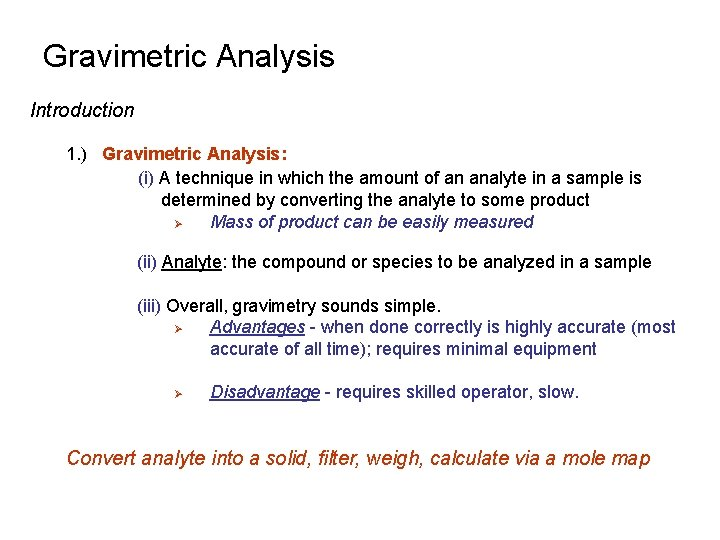Gravimetric Analysis Introduction 1. ) Gravimetric Analysis: (i) A technique in which the amount of an analyte in a sample is determined by converting the analyte to some product Ø Mass of product can be easily measured (ii) Analyte: the compound or species to be analyzed in a sample (iii) Overall, gravimetry sounds simple. Ø Advantages - when done correctly is highly accurate (most accurate of all time); requires minimal equipment Ø Disadvantage - requires skilled operator, slow. Convert analyte into a solid, filter, weigh, calculate via a mole mapGravimetric Analysis Introduction 1. ) Gravimetric Analysis: (iii) Example: Ø Determination of lead (Pb+2) in water Pb+ Analyte Ø Ø + 2 Cl- Reagent Pb. Cl 2(s) Solid Product By adding excess Cl- to the sample, essentially all of the Pb+2 will precipitate as Pb. Cl 2. Mass of Pb. Cl 2 is then determined. - used to calculate the amount of Pb+2 in original solution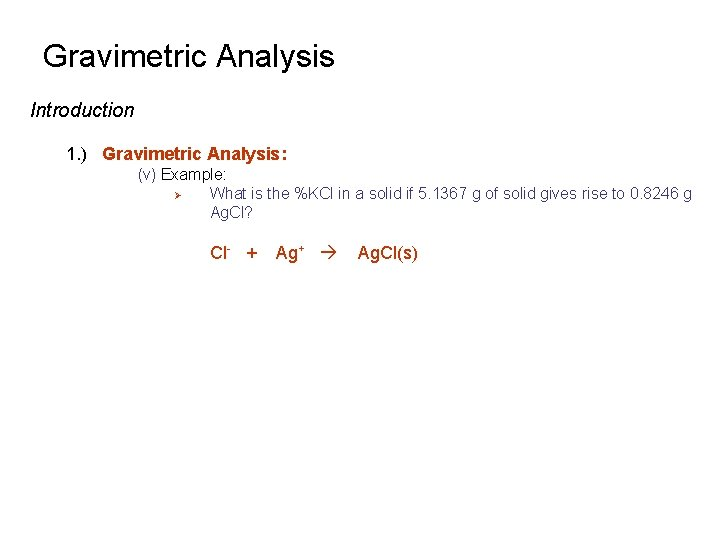Gravimetric Analysis Introduction 1. ) Gravimetric Analysis: (v) Example: Ø What is the %KCl in a solid if 5. 1367 g of solid gives rise to 0. 8246 g Ag. Cl? Cl- + Ag+ Ag. Cl(s)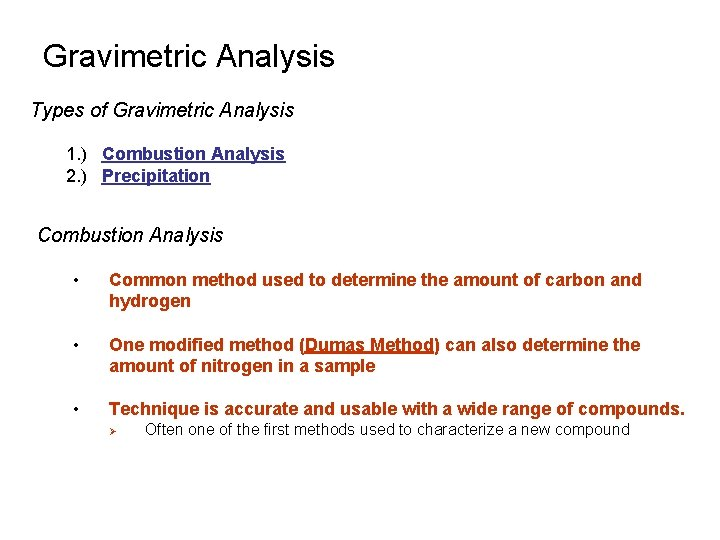Gravimetric Analysis Types of Gravimetric Analysis 1. ) Combustion Analysis 2. ) Precipitation Combustion Analysis • Common method used to determine the amount of carbon and hydrogen • One modified method (Dumas Method) can also determine the amount of nitrogen in a sample • Technique is accurate and usable with a wide range of compounds. Ø Often one of the first methods used to characterize a new compoundGravimetric Analysis Combustion Analysis 1. ) Principals: (i) Sample is heated in presence of Oxygen (O 2) Ø Converts carbon in sample to CO 2 Ø Converts hydrogen in sample to H 2 O C(sample) + O 2 2 H(sample) + ½O 2 D Pt CO 2 H 2 O Pt, Cu. O, Pb. O 2, or Mn. O 2 is used as a catalyst in this process (ii) As CO 2 and H 2 O form, leave the sample and flow through a series of chambers Ø Chambers contain chemicals that bind one or both of these products Ø Example: - P 4 O 10 can be used to absorb H 2 O Ø Ascarite - Ascarite can be used to absorb CO 2 - Ascarite - Sodium Hydroxide Coated Non-Fibrous Silicate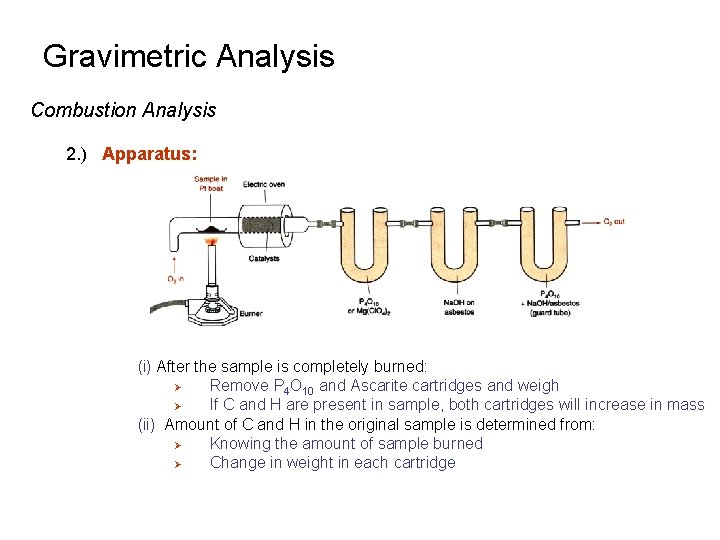Gravimetric Analysis Combustion Analysis 2. ) Apparatus: (i) After the sample is completely burned: Ø Remove P 4 O 10 and Ascarite cartridges and weigh Ø If C and H are present in sample, both cartridges will increase in mass (ii) Amount of C and H in the original sample is determined from: Ø Knowing the amount of sample burned Ø Change in weight in each cartridge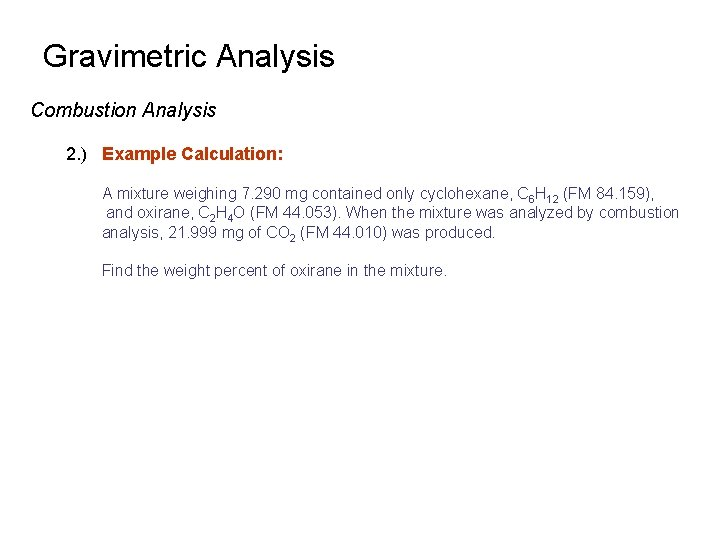Gravimetric Analysis Combustion Analysis 2. ) Example Calculation: A mixture weighing 7. 290 mg contained only cyclohexane, C 6 H 12 (FM 84. 159), and oxirane, C 2 H 4 O (FM 44. 053). When the mixture was analyzed by combustion analysis, 21. 999 mg of CO 2 (FM 44. 010) was produced. Find the weight percent of oxirane in the mixture.Gravimetric Analysis Precipitation Analysis 1. ) Principals: (i) Reagent + Analyte Solid Product (collect and measure mass) (ii) Desired Properties of Solid Product Ø Should be very insoluble Ø Easily filterable (i. e. , large crystals) Ø Very Pure Ø Known and constant composition Few precipitates have all of these properties, but in most cases appropriate techniques can help optimize these qualities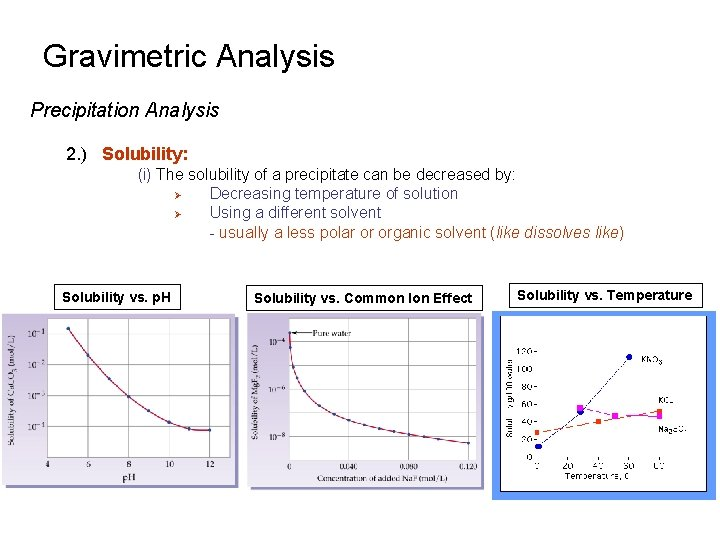Gravimetric Analysis Precipitation Analysis 2. ) Solubility: (i) The solubility of a precipitate can be decreased by: Ø Decreasing temperature of solution Ø Using a different solvent - usually a less polar or organic solvent (like dissolves like) Solubility vs. p. H Solubility vs. Common Ion Effect Solubility vs. TemperatureGravimetric Analysis Precipitation Analysis 1. ) Gravimetric Analysis: (vi) Governed by equilibrium: Ag. Cl Ksp = 1. 8 x 10 -10 Solubility of Ag. Cl = [Ag+] + [Ag. Cl 2 -] Cl- + Ag+ Ag. Cl(ag) ion pair formation Ag. Cl(aq) Ag. Cl(s) intrinsic solubility Ag. Cl +Cl- Ag. Cl 2 - complex ion formation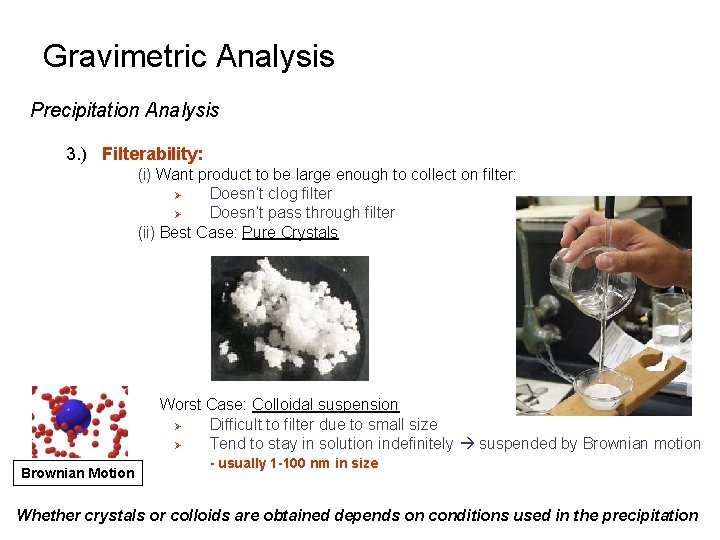Gravimetric Analysis Precipitation Analysis 3. ) Filterability: (i) Want product to be large enough to collect on filter: Ø Doesn’t clog filter Ø Doesn’t pass through filter (ii) Best Case: Pure Crystals Worst Case: Colloidal suspension Ø Difficult to filter due to small size Ø Tend to stay in solution indefinitely suspended by Brownian motion Brownian Motion - usually 1 -100 nm in size Whether crystals or colloids are obtained depends on conditions used in the precipitation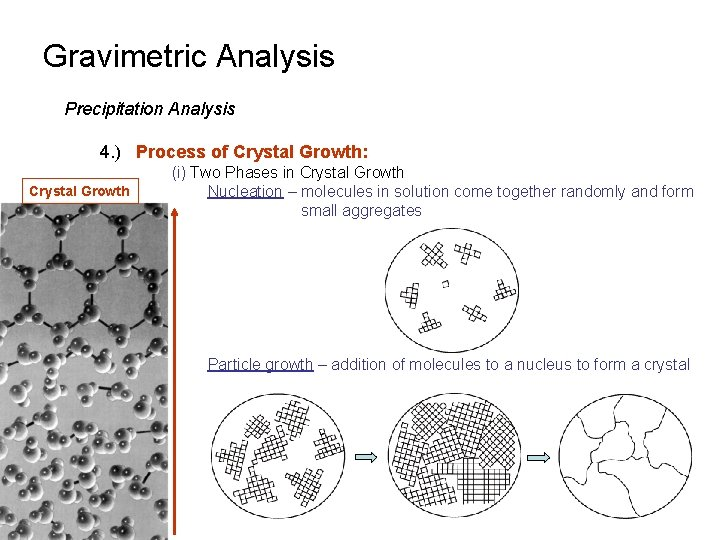Gravimetric Analysis Precipitation Analysis 4. ) Process of Crystal Growth: Crystal Growth (i) Two Phases in Crystal Growth Nucleation – molecules in solution come together randomly and form small aggregates Particle growth – addition of molecules to a nucleus to form a crystalGravimetric Analysis Precipitation Analysis 4. ) Process of Crystal Growth: (ii) Nucleation and Particle growth always compete for molecules/ions being precipitated. Ø If nucleation is faster than particle growth: - a large number of small aggregates occur giving colloidal suspensions Ø If particle growth is faster than nucleation: - only a few, large particles form giving pure crystals Want to Convert to Colloidal suspension Crystal formationGravimetric Analysis Precipitation Analysis 4. ) Process of Crystal Growth: (iii) Rates of nucleation vs. particle growth depends on: Ø Amount of precipitation solute present Ø Described by a quantity known as the Relative Supersaturation (R) S = concentration of solute in solution at equilibrium Q = actual concentration of solute added to solution Colloidal Particle (iv) If R is large, Ø Large relative amount of solute in solution Ø Favors nucleation and colloid formation (v) In gravimmetry based on precipitations, a small value of R (~1. 0) is desired in order to favor large crystal growth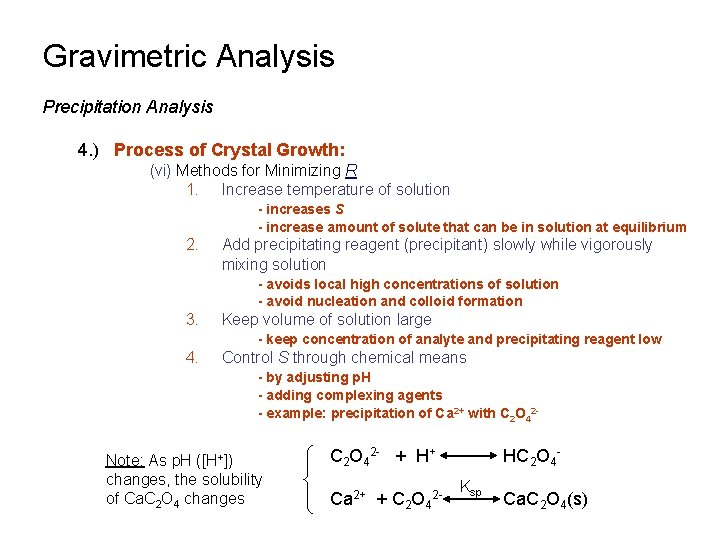Gravimetric Analysis Precipitation Analysis 4. ) Process of Crystal Growth: (vi) Methods for Minimizing R 1. Increase temperature of solution - increases S - increase amount of solute that can be in solution at equilibrium 2. Add precipitating reagent (precipitant) slowly while vigorously mixing solution - avoids local high concentrations of solution - avoid nucleation and colloid formation 3. Keep volume of solution large - keep concentration of analyte and precipitating reagent low 4. Control S through chemical means - by adjusting p. H - adding complexing agents - example: precipitation of Ca 2+ with C 2 O 42 - Note: As p. H ([H+]) changes, the solubility of Ca. C 2 O 4 changes C 2 O 42 - + H+ Ca 2+ + C 2 O 42 - HC 2 O 4 Ksp Ca. C 2 O 4(s)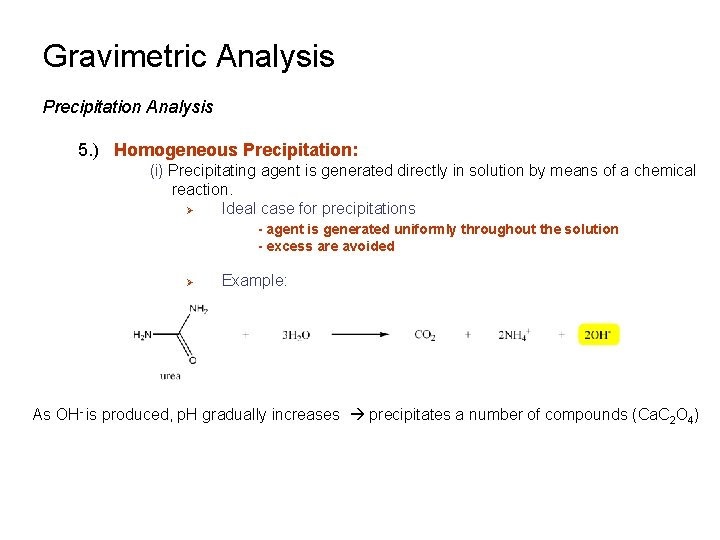Gravimetric Analysis Precipitation Analysis 5. ) Homogeneous Precipitation: (i) Precipitating agent is generated directly in solution by means of a chemical reaction. Ø Ideal case for precipitations - agent is generated uniformly throughout the solution - excess are avoided Ø Example: As OH- is produced, p. H gradually increases precipitates a number of compounds (Ca. C 2 O 4)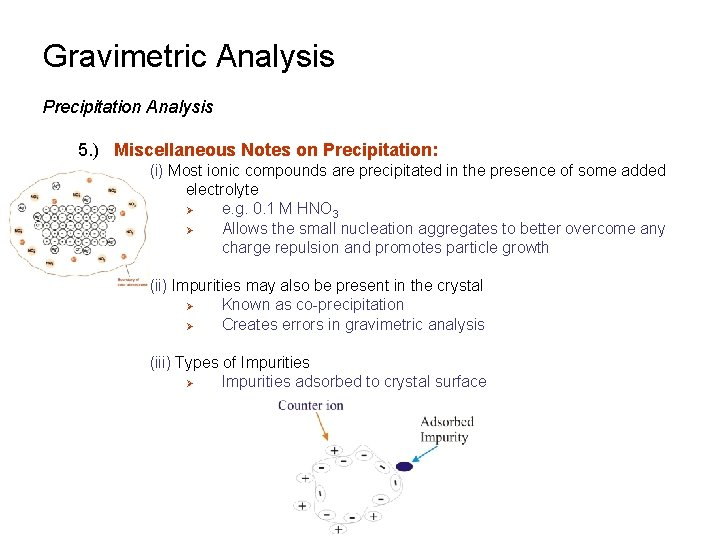Gravimetric Analysis Precipitation Analysis 5. ) Miscellaneous Notes on Precipitation: (i) Most ionic compounds are precipitated in the presence of some added electrolyte Ø e. g. 0. 1 M HNO 3 Ø Allows the small nucleation aggregates to better overcome any charge repulsion and promotes particle growth (ii) Impurities may also be present in the crystal Ø Known as co-precipitation Ø Creates errors in gravimetric analysis (iii) Types of Impurities Ø Impurities adsorbed to crystal surfaceGravimetric Analysis Precipitation Analysis 5. ) Miscellaneous Notes on Precipitation: (iii) Types of Impurities Ø Impurities absorbed or trapped within pockets in the crystal Ø Occlusion Ø Ø Impurities similar to the analyte or reagent Impurities placed in the crystal instead of analyte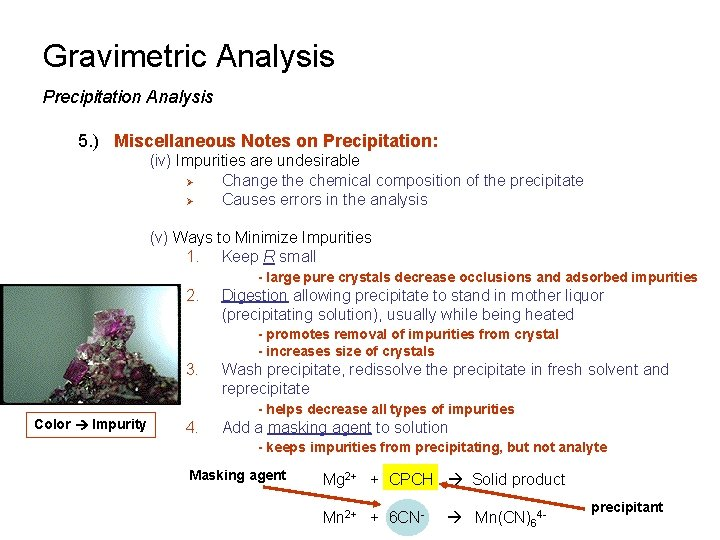Gravimetric Analysis Precipitation Analysis 5. ) Miscellaneous Notes on Precipitation: (iv) Impurities are undesirable Ø Change the chemical composition of the precipitate Ø Causes errors in the analysis (v) Ways to Minimize Impurities 1. Keep R small - large pure crystals decrease occlusions and adsorbed impurities 2. Digestion allowing precipitate to stand in mother liquor (precipitating solution), usually while being heated - promotes removal of impurities from crystal - increases size of crystals 3. Wash precipitate, redissolve the precipitate in fresh solvent and reprecipitate - helps decrease all types of impurities Color Impurity 4. Add a masking agent to solution - keeps impurities from precipitating, but not analyte Masking agent Mg 2+ + CPCH Solid product Mn 2+ + 6 CN- Mn(CN)64 - precipitant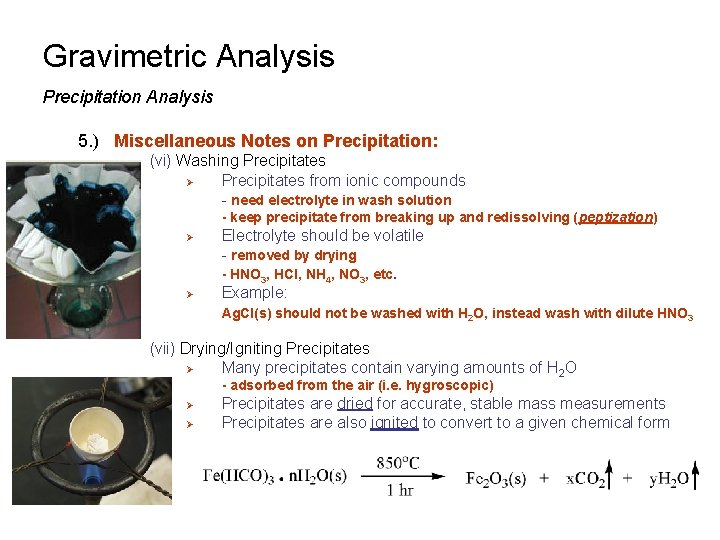Gravimetric Analysis Precipitation Analysis 5. ) Miscellaneous Notes on Precipitation: (vi) Washing Precipitates Ø Precipitates from ionic compounds - need electrolyte in wash solution - keep precipitate from breaking up and redissolving (peptization) Ø Ø Electrolyte should be volatile - removed by drying - HNO 3, HCl, NH 4, NO 3, etc. Example: Ag. Cl(s) should not be washed with H 2 O, instead wash with dilute HNO 3 (vii) Drying/Igniting Precipitates Ø Many precipitates contain varying amounts of H 2 O - adsorbed from the air (i. e. hygroscopic) Ø Ø Precipitates are dried for accurate, stable mass measurements Precipitates are also ignited to convert to a given chemical formGravimetric Analysis Scope of Gravimetric Analysis 1. ) Accurate 2. ) Inexpensive Ø Only major equipment is balance 3. ) Method is more tedious than other approaches Ø must carefully consider how to minimize potential interferences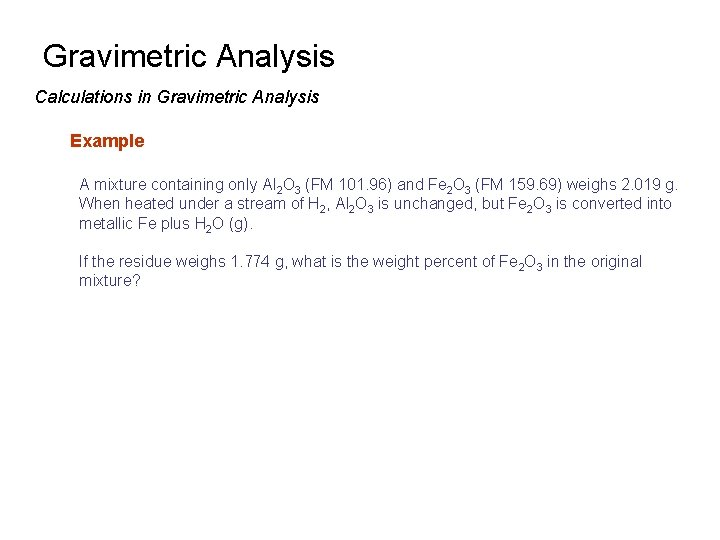Gravimetric Analysis Calculations in Gravimetric Analysis Example A mixture containing only Al 2 O 3 (FM 101. 96) and Fe 2 O 3 (FM 159. 69) weighs 2. 019 g. When heated under a stream of H 2, Al 2 O 3 is unchanged, but Fe 2 O 3 is converted into metallic Fe plus H 2 O (g). If the residue weighs 1. 774 g, what is the weight percent of Fe 2 O 3 in the original mixture?